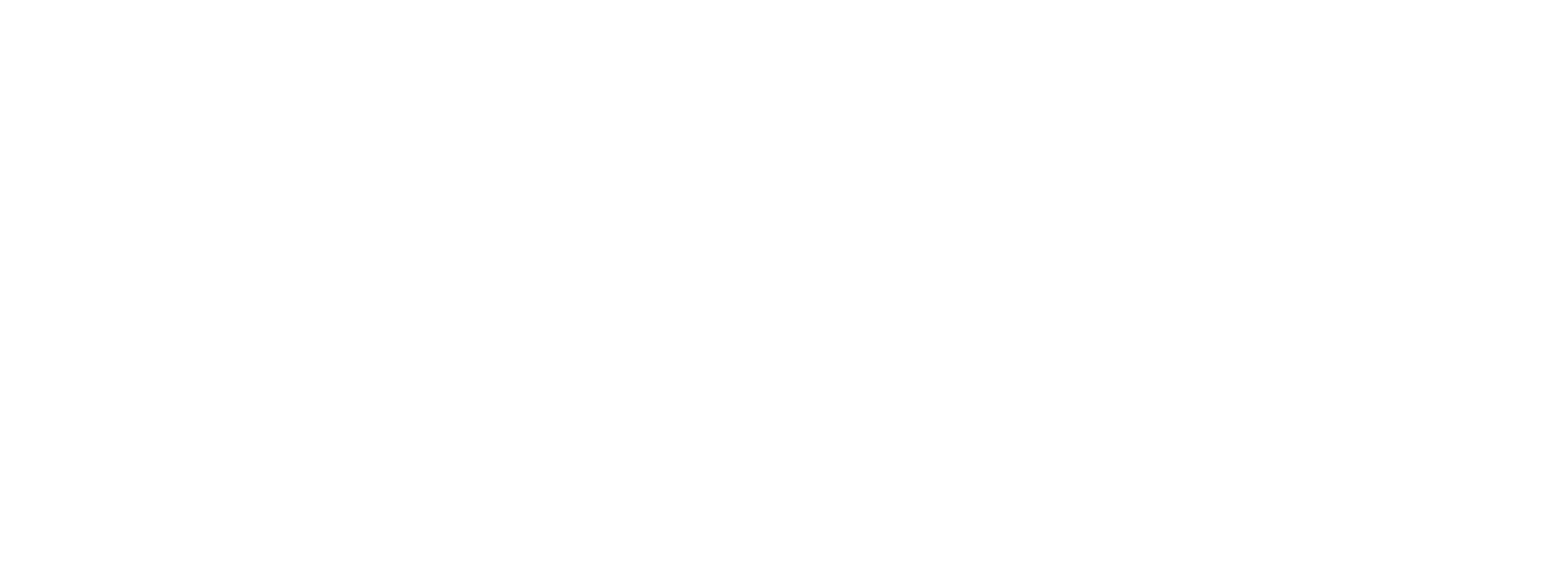# Interview Question For R programming

0
310## Introduction To Interview Question For R programming

1. What are the different types of data structures in R?
• Vector
• Matrix
• List
• DataFrame
• Vector:- vector is the store sequence of elements of the same data type values. Member in the vector are called as component
• Matrix:- Matrix is the 2D Data structure store values in rows and columns format. You can bind the two different matrix in row-wise or column-wise.
• List:- To store value Sequence of element in different data type like number string vector etc.
• Dataframe:-  DataFrame is more advance that Matrix is used to store different data type values in different columns and it combines the features of matrix and list
1. How to install package in R?

The below command is used to install a package in R:

install.packages(“<package_name>”)

1. Name some packages in R, which can be used for data imputation?

Following are some package is used for data imputation

• Amelia
• missForest
• Hmisc
• Mi
• imputeR
``
1. Explain about the confusion matrix in R?

The confusion matrix is used to evaluate the classification type of model. It calculate cross-tabulation between Actual and the predicted value.for to calculate confusion matrix use caTools package and use confusion matrix() function

1. Syntax to define function in R?

To create function in R use function keywords

Function_name <- function( arguments1, argument2) {

Function Body

}

1. What are the different functions in “dplyr” package?
• filter
• select
• mutate
• arrange
• Count

7. What is the advantage of using an apply family of functions in R?

Apply function is used to applies some function in data frame values and matrix values in R.

Syntex:-

apply(var, margin, Function)

Var is array or matrix

Margin used to decise the function is applieds on row(margin=1) or column(margin=2) or both margin=c(1,2).

8. Data mining packages in R?

1. rpart and caret package:- For machine learning model
2. Data.table:- to reading large files
3. Arules :- assod=ciation rule learning
5. tm:- for text mining
6. Forecast:- for time series analysis

9. What are the uses of rbind and cbind function in R?

rbind is used for row adding in maxis or DataFrame condition in data frame columns name are the same both data frame to join.

Cbind is used for adding columns in the matrix and the data frame, the condition is dimensions of both the data frame is the same.

``

10. How would you make multiple plots onto a single page in R?

Plotting a multiple graph in a single page is to use par function nad pass plot dimension  like par(mfrow=c(2,2))

1. hist(iris\$sepal.Length)
2. hist(iris\$sepal.Width)
3. hist(iris\$Petal.Length)
4. hist(iris\$Petal.Width)

11. How to plot a scatter plot using the GGplot2 package?

To make a scatter plot using ggplot pass both numerical variables in X and Y axis it shows the relationships within data.

Ex

ggplot(iris,aes(y=Sepal.Length,x=Petal.Length))+geom_point()

12. How would you do a left and right join in R?

To use the “dplyr” package to make a left and right join.

Use left join function collect all data from left data frame

left_join( Data_frame1,Data_frame2,by=’Column_name’)

By = pass same column name to join

Use Right join collect all data from right data frame

right_join(Data_Frame1,Data_Frame2,by= “Column_name”)

13.  What is a factor? How would you create a factor in R?

Factors are the variable in R which takes categorical variables into numerical variable. we are set levels and labels of factor to get statistical analysis of categorical variables.

14. How would you join multiple strings together?

To join string in R using paste() in R orstring_c() from stringR package

paste(“Fruit”,”Apple”)

str_c(“Fruit”,”Apple”, sep=”-“)

``

15. What are the different import functions in R?

Different types of file we import function in R

The following are some import file functions.

16. Which function is used to debugging in R?

The following are the debugging functions.

• traceback()
• debug()
• browser()
• trace()
• recover()

17. How to identify the count of different categorical variables in R?

using table function you get the idea about the distribution of categorical variable in R

table(iris\$species)

For percentage

table(iris\$species)/nrow(iris)

18. How would you find the number of missing values in a dataset and remove all of them?

To check the number of null values in data frame

sum(isna(datframe name))

to drop use omit function

no.omit(datafarme_name)

19. How would you measure correlation in R?

Cor Function is used to check correlation in R

Cor(Column name)

20. How would you rename the columns of a data frame?

To rename Column name use colnames function

colnames(dataframe_name) <- C( New Column name)